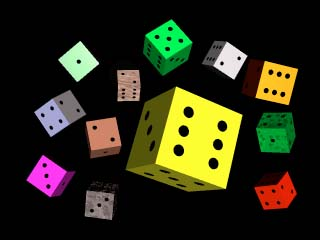# Fast Dice Statistics in C

## And Java!Once upon a flame war, to win a debate on which Hero System attack was more cost effective, I needed complete statistics for chances of rolling a given number on twelve six sided dice. My opponent, a university professor, had used a large number of random number generator calls to build his bell curves and generate his statistics. I decided to one up him.

First thing I tried was to build twelve nested for loops and generate a brute force solution. Unfortunately, it would have taken my poor Apple II a few months to work it's way through the loops.

My second attempt was to buy a book on statistics. Unfortunately, it would have taken me a few months to work my way through the loops.

The following links lead to C versions of my third and fourth attempts. Both count on the same principle. If you know the probabilities of rolling N dice, calculating the table for N+1 is quick and easy. (The number of ways to roll X on N dice with S sides each is the sum (i from one to S) of the ways to roll X - i on N - 1 dice.) The first implementation is based on recursive logic. It is easier to see the algorithm in the recursive version, but recursive programming makes many people dizzy.

The second implementation uses three nested loops to solve the same problem, and stores the results in a two dimensional array. The array contains the number of ways with a given number of dice to roll a given number. Ways[number of dice][roll this number].

To get the probability of rolling a given number, divide the number of ways to roll a number by the number of sides on your dice raised to the power of the number of dice. Thus, for three six sided dice, divide by six to the third power, or 216.

Do not input negative numbers into the recursive version. Result will not be meaningful. If you need a complete table of probabilities, the loop version will run faster. However, the recursive version might be more flexible if you are changing the number of dice or the number of sides within your program.

Recursive - The method coded into a recursive C function.

Three Loop - The method coded into a traditional C function.

Java - A (hopefully) working Java applet implementation of the Three Loop method. Nescape 4.0 or Internet Explorer 4.0 recomended.

Java Statisics Class - Half the code used for the above applet.

Java Applet Class - The user interface stuff. This has nothing to do with dice statistics, but everything to do with object oriented, event driven, write once run anywhere programming. If you think you know how to program, you might want to take a peek at Java, not that this is the best example.## Warp Nine Flying False Colors!